## Sample routine of working with LPT1 port under windows XP in CPP language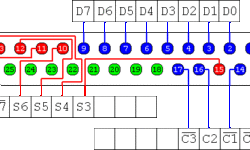This is a simple routine of sending and reading a byte from the LPT1 port under Windows XP. LPT port has four types of pins: 8 output pins accessed via the DATA Port 5 input pins (one inverted) accessed via the STATUS Port 4 output pins (three inverted) accessed via the CONTROL Port The remaining 8 pins are grounded Now we are interested in Data pins.

## Variables in embedded C programming language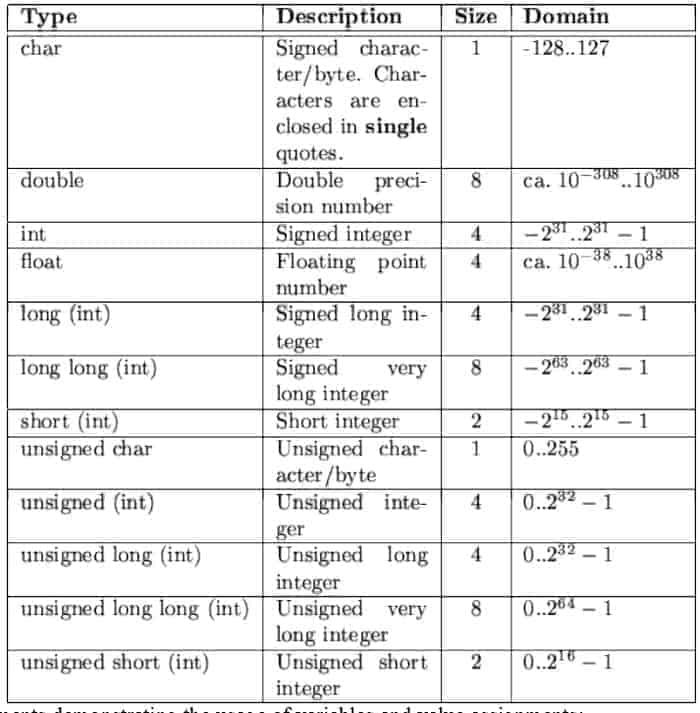What are variables in the C language? Variables are simple keywords that are defined by the values. Values can be changed. Variables are like a box with some size where values like apples can be put in. So variables can be various forms and sizes, so-called variable types. Variable type is defined by a reserved word which indicates the type and size of variable identifier: unsigned char my_char; long int all_my_numbers; int number; Why do we need variables? The basic answer is that memory is limited, and the compiler needs to know much space to reserve for each variable. The coder needs to specify the variable type and its size by using one of the reserved words from the table:

## Gauss-Zeidel optimization routines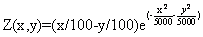This is the simplest optimization routine. Using this algorithm, optimization parameters are changed separately in each step. Only one parameter can be changed in one step while others are held as constants. Xk+1=Xk+ΔXk , k=0,1,2,… ΔXk step of parameter Xk. The parameter is changed until function growth is noticed, and then the next parameter follows, and so on. After the cycle with all parameters is completed, the step is changed to half its value and repeats. Optimal point searching ends when there is no function increase, and the last point is held as an optimal point. First function optimization example Its plot: Using the MATLAB script, we get the results below. In each picture, the start coordinates are different.

## Impulse Response of discrete system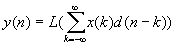Impulse signal can be represented as:d[n] = 1, if n=0d[n] = 0, otherwiseit can also be written like d=[1,0,0,0,â€¦] Impulse Response The impulse response h(n) is the response of filter L() at time n to unit impulse occurring at time 0.h(n)=L(d(n))Let’s see how a discrete system can be described when impulse response is known We know that: In the linear system this can be written as follows: Because h(n-k)=L(d(n-k))Then: What do we get? Its impulse response can describe a linear system. The last expression is called convolution. This is the heart of DSP Filtering.To write this sum in the more convenient matter is assumed that: Matlab example Matlab example: As you can see if the input queue has N samples, impulse response has M after convolution there will be total N+M-1 samples.

## Pointers to structures in embedded CSometimes you might like to manipulate the members of C structures in a more generalized way. This can be done by using pointer reference to structure. Normally it is easier to pass a pointer to a structure than passing the entire structure to function. Structure pointer can be declared very easily: For instance: we can assign the first structure address to the structure pointer like this: now we can access the first structure members by using structure pointer: or we can write Structures can contain member pointers to other structures or structures of the same type. This way, we can create lists – dynamic structure: This way, you can assign the pointer with the address to another structure: Read more about dynamic structures in C tutorials on the internet.

## C language Operators and expressions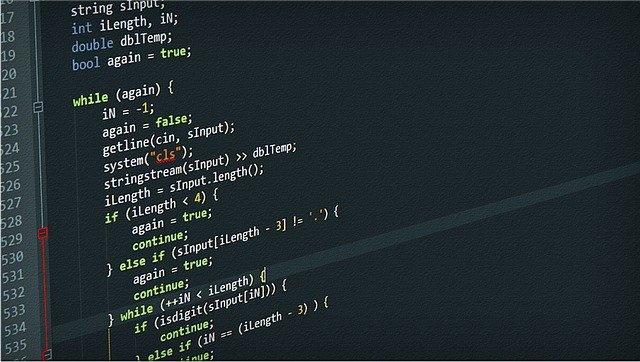The main thing that microcontrollers does is operates with data. Microcontrollers do four main operations: adds, abstracts, multiplies, and divides (+,-,*,/). division can be split into the division “/” and modulus operation “%.” For instance, i1/i2 is an integer division. Another part of the operators is Relation operators. They are used for boolean conditions and expressions. Expressions with these operators return true or false values. Zero is taken as false and non zero value as true. Operators may be as follows: <, <=, > >=, ==, !=. The priority of the first four operators is higher than that of the latter two operators. These operators are used in relational expressions such as: Note that the equality operator is == and not =. ‘=’ is an assignment operator. If you want to compare a and b for equality, then you should write a == b, not a = b because a = b means you are assigning the value of b to a, as shown in.

## Constants in C languageConstant value is understandable as nonchangeable value like PI=3.141592… value in math. Usually, you use constants in your programs, but don’t realize that they are constants. For instance: x=x+3; The number 3 is a constant which will be compiled directly in addition operation. Constants can be character or string. Like in function printf(“Hello World\n”); “Hello World” is a string constant that is placed in program memory and will never change. It is usually recommended to declare constants by using identifier with reserved word const: const int No=44; Identifying the variable as constant will cause the compiler to store this variable in program memory rather than in RAM, thus saving space in RAM. If special functions are used, then constants can also be stored in EEPROM memory.

## The very basics of CC language is a function-based programming language. C program itself is a function. Usually, parameters to the C function are passed as arguments. The function consists of a name followed by the parentheses enclosing arguments or an empty pair of parentheses if there are no arguments required. If there are several arguments, they are separated by commas. The mandatory part of the C program is the main function. This function must be included in every program because it is the first function run after its execution. Lets take an example: This is an elementary C program, but it contains all the necessary elements. Let’s examine a little bit about what we have written here…